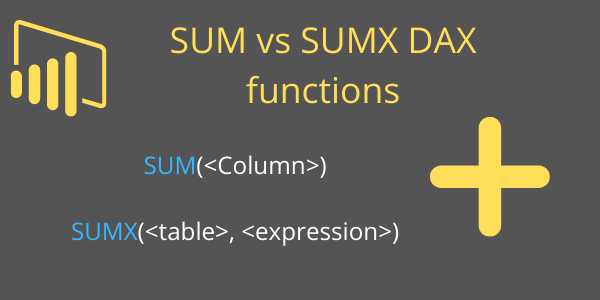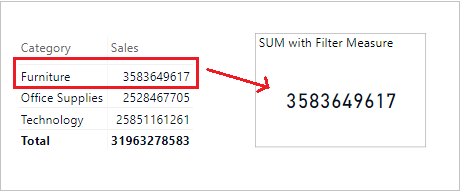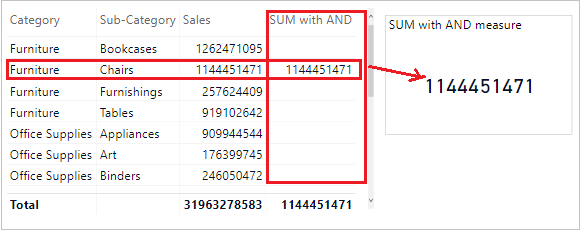Home » DAX » DAX SUM and SUMX Functions

# DAX SUM and SUMX FunctionsThe Power BI DAX functions SUM & SUMX both are aggregation functions and comes under Aggregation functions Dax categories.

some important DAX functions:- CALCULATE & Filter

### 1- SUM DAX function

The SUM function is a aggregation function and it calculates the sum of all numbers in a column.

#### Syntax:

`SUM(<Column>)`

#### Description:

 S no. Parameter Description 1 Column The column that contains the numbers to sum.

Note: SUM support only single argument.

#### Let’s understand with an example:

Step-1: Create a measure for SUM function

`TotalSales = SUM('Global-Superstore'[Sales])`

Step-2: Now drag “TotalSales” measure to card visual to see the output of sales measure.SUM DAX

As you see in above screen shot, SUM measure returns the total summation of Sales column.

#### SUM function with Filter

Step-1: Create a measure to get the sales of “Furniture” category.

`SUM with Filter =CALCULATE( SUM('Global-Superstore'[Sales]),FILTER('Global-Superstore', 'Global-Superstore'[Category]="Furniture"))`

Step-2: Output of above measure.SUM with Filter

#### SUM DAX with AND function

Step-1: Get the “Furniture” category sales where “Sub category” is chairs.

`SUM with AND = CALCULATE(SUM('Global-Superstore'[Sales]),FILTER ('Global-Superstore',AND ('Global-Superstore'[Category] = "Furniture",'Global-Superstore'[Sub-Category]="Chairs")))`

Step-2: Drag measure to Table & Card visual, and it will return the sum only for whether both conditions are true.SUM with AND function

#### SUM DAX with OR function

`SUM with OR = CALCULATE(SUM('Global-Superstore'[Sales]),FILTER ('Global-Superstore', OR ('Global-Superstore'[Category] = "Furniture", 'Global-Superstore'[Sub-Category]="Chairs")))`

It will return SUM of sales whether one condition true.SUM with OR function

### 2- SUMX DAX function

SUMX is an iterator function. Returns the sum of an expression evaluated for each row in a table. With this function you can operate on multiple columns in table row wise.

#### Syntax:

`SUMX(<table>, <expression>)`

#### Description:

 S no. Parameter Description 1 Table The table containing the rows for which the expression will be evaluated. 2 expression The expression to be evaluated for each row of the table.

Get Total sales to using SUMX function:

`Total Sale = SUMX('Global-Superstore', 'Global-Superstore'[Sales])`

### Some more scenario with SUMX functions :-

1- Suppose you want to see row wise sum of “Sales” & “Profit” columns together. Here, SUMX helps you because it is iterator function and perform the operation row wise.

`Sales + Profit =SUMX('Global-Superstore' , 'Global-Superstore'[Sales] + 'Global-Superstore'[Profit])`SUMX DAX

#### 2- Get sales of particular year

`2013 Sales =SUMX (FILTER ( 'Global-Superstore', Year('Global-Superstore'[Order Date]) = 2013 ), 'Global-Superstore'[Sales])`

#### 3- SUMX with AND function

`SUMX with AND = SUMX(FILTER ('Global-Superstore', AND ( 'Global-Superstore'[Category] = "Furniture", 'Global-Superstore'[Sub-Category]="Chairs")), 'Global-Superstore'[Sales])`

#### 4- SUMX with OR function

`SUMX with OR = SUMX(FILTER ('Global-Superstore', OR ('Global-Superstore'[Category] = "Furniture", 'Global-Superstore'[Sub-Category]="Chairs")), 'Global-Superstore'[Sales])`SUMX with AND-OR function

Refer more DAX functions: DAX functions

Hope you enjoyed the post. Your valuable feedback, question, or comments about this post are always welcome or you can leave us message on our Contact form , we will revert to you asap.